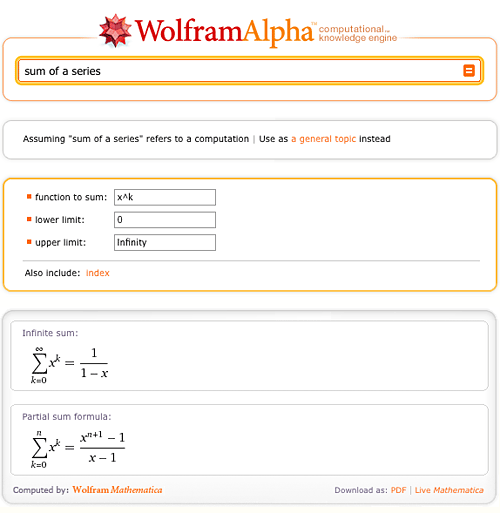# Yes, Those Calculators Are Dynamic

November 1, 2010 —Comments Off

Wolfram|Alpha is still young and constantly improving. One of the biggest hurdles that our developers are constantly faced with is how to correctly interpret the meaning of general user inputs like “How do I factor an equation?”

Wolfram|Alpha is great at calculating answers that have specific inputs, but when general concepts are given (like “factoring”, for example), it becomes a bit tricky. How would one calculate a concept like that? Let us look at a specific example—Wolfram|Alpha can easily calculate integrals, as long as you ask it to integrate an explicit function. But what happens when you simply ask Wolfram|Alpha to “integrate”? Before, had you given this input, a number of examples using the word “integrate” would have been generated to show how to properly ask Wolfram|Alpha to calculate an integral. But now, when you enter a generic term or question related to a specific math function or formula, it provides a simple query-specific calculator.

For example, given the query “Show me how to integrate”, the following results appear:

Notice that new input fields appear (as well as optional ones in case the integral is to be calculated within a range, and/or with multiple variables). Similarly, notice how the input fields differ depending on the query:

In the example above, there are two input fields: one for the unit and value that are being converted, and the other field for the unit which it will be converted to.

In the example above, there are two input fields for two different dates. Wolfram|Alpha does the rest and calculates the difference between the two.

In the example here, you are able to ask Wolfram|Alpha to calculate the sum of any series you want.And in this final example, we demonstrate how Wolfram|Alpha can plot equations, as well as graph them in the domain you desire by clicking “plot range of variable”.

These are just a few examples of the dynamic calculators available in Wolfram|Alpha. You can also find calculators that calculate derivatives, Taylor series, Fourier series, products, sums, as well as calculators that find factors, solve for variables of an equation, and plot the midpoint of a line segment—the list is constantly growing! What other types of dynamic calculators would you like to see in Wolfram|Alpha?

Typo in Line 11 of the text above. ‘enters’ for ‘enter’
Brian Gilbert, Volunteer Curator

Posted by Brian Gilbert November 1, 2010 at 12:48 pm

Hello Brian,

Thank you for bringing this to our attention; it has been fixed!

The Wolfram|Alpha Team

Posted by The PR Team November 1, 2010 at 10:12 pm

hi!!! this is wonderfull!!!! keep it up guys!!

Posted by sitanshu November 2, 2010 at 1:03 am

I absolutely love this site! It always helps me when I’m stumped on mathematical equations. Keep up the great work!!

Posted by Heidi Landsmann November 2, 2010 at 1:04 pm

This is really cool, I like this.

Posted by Eric November 4, 2010 at 12:00 am

What happened to “Show steps” link? it doesn’t show the solutions anymore? is it being disabled or it’s just a glitch? Before, it helped me a lot with my calculus misunderstandings in class…

Posted by Max November 8, 2010 at 11:58 pm

Hi Max, Thank you for bringing this to our attention. Our team is working on a fix. Hopefully it will be back up in time to tackle more calculus! Thank you.

Posted by The Wolfram|Alpha Team November 9, 2010 at 12:06 am

Perhaps Wolfram could use contextual information to interpret the meaning of a phrase including a word like ‘factor’. There are so many websites with applications and applications and hybrids – I can see a future in which search engines will perform all these functions, rather than just suggesting websites that do.

Posted by Ben Acheson November 10, 2010 at 4:36 am